# Black-Scholes Pricing Model and Assumptions

Pricing Model: Carta uses the Black-Scholes Formula that includes the below assumption calculations.

Expected Term: The expected term assumption in the Black-Scholes is intended to represent the average time the Company expects the option grant to remain outstanding before it is either exercised or forfeited. Due to the lack of historical data captured by many nonpublic entities, ASC 718-10-30-20A allows nonpublic entities to elect to use the practical expedient to estimate the expected term. This means non-public entities can use the midpoint between the requisite service period and the contractual term of the award. Carta uses this method to calculate the expected term for grants issued to employees. This calculation is applied to each individual grant uniquely by using the grant date, vesting schedule, and grant term. To measure this for a single grant, Carta uses the following formula: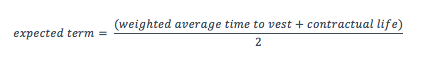Additionally, according to ASC 718-10-30-10A Companies can elect to use the contractual term as the expected term for non-employee awards. As a result, Carta calculates the expected term for non-employee option grants as follows: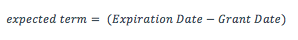Interest Rate: For the interest rate calculation, Carta downloads and maintains the daily Treasury Constant Maturities Rates provided by the Federal Reserve (http://www.federalreserve.gov/releases/h15/data.htm). Based on the expected term of each individual grant, the associated rate is used. In the event the grant’s expected term does not match to an interest rate period provided by the Federal Reserve, Carta will do a weighted calculation using a compounded floor and ceiling rate (compounded to account for the semiannual coupon). The floor and ceiling rates are determined by the two periods that are closest to the expected term value.

For example, let’s look at an award granted on February 1, 2014 with a 6.3 year Expected Term. The following is a list of the 5-year Treasury Yields and the 7-year Treasury Yields on January 29th, 30th, 31st, and February 3rd of 2014.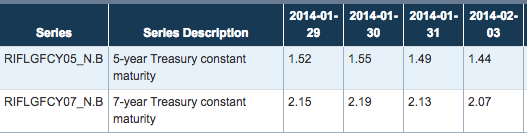Since the Federal Reserve does not publish interest rates for a 6.3-year bond, Carta interpolates the 5-year and the 7-year rates to estimate what the 6.3-year rate would be. From here, Carta assumes these rates to be the stated annual rates and converts these rates into continuously compounded annual effective rates using the following formulas: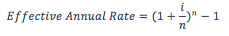*Where i is the stated annual rate listed above and is the number of compounding periods.

Since Treasury bond coupons are paid semi-annually, the stated annual rate includes 2 compounding periods each year. Using the 5-year rate on January 31, 2014 of 1.49% and the 7-year rate on January 31, 2014 of 2.13, their effective annual rates are 1.4956% and 2.1413%, respectively. The effective annual rates are then continuously compounded: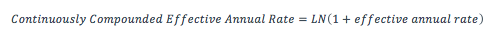Once done, the rates we interpolate will be 1.4845% and 2.1187% for the 5-year and 7-year terms. We then interpolate the two rates to estimate the 6.3-year term using this formula: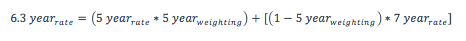The resulting interest rate used would then be 1.8967%.

Volatility: To begin the volatility calculation, Carta requires an input of a set of Peer Companies provided by the Company’s administrator generating the expense reports. Once this input is collected, Carta calculates the volatility for each individual grant. This is done by first calculating the standard deviation of the change in the natural log of the daily closing price of each individual peer company using the grant’s issue date as a starting point over a period covering the expected term for the amount of data available. This is done for each individual peer company and averaged evenly to determine the ultimate assumption used for that grant. All historical data is pulled from a third party data provider (https://www.quandl.com).

Dividend: Carta assumes that the dividend is zero.

Fair Market Value: This is provided by the company on a Fair Market Value table, before reports are ran in Carta. We take the value from this table based on the Fair Market Value on the grant date.

Was this article useful? Thanks for the feedback There was a problem submitting your feedback. Please try again later.